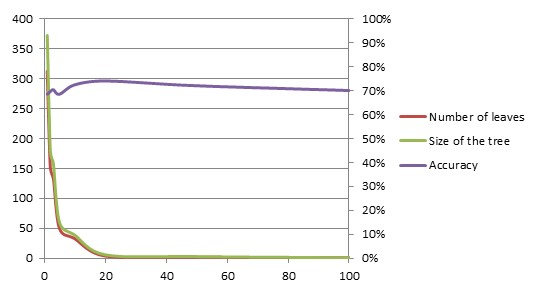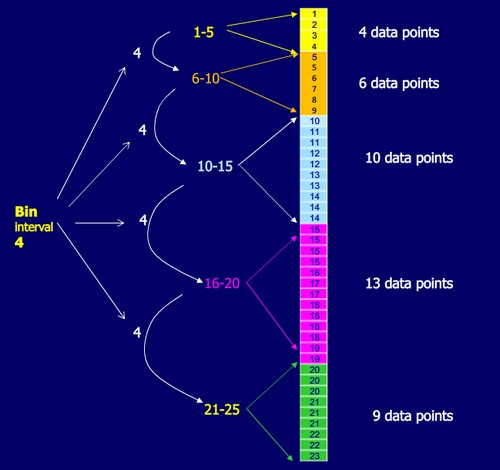# Data Mining - Information Gain

Information theory was find by Claude Shannon. It has quantified entropy. This is key measure of information which is usually expressed by the average number of bits needed to store or communicate one symbol in a message.

Information theory measure information in bits

$entropy(p_1,p_2,\dots,p_n)=-{p_1}log(p_1)-{p_2}log(p_2)-\dots-{p_n}log(p_n)$

Information gain is the amount of information gained by knowing the value of the attribute

$\text{Information gain} = \text{(Entropy of distribution before the split)} – \text{(entropy of distribution after it)}$

Information gain is the amount of information that's gained by knowing the value of the attribute, which is the entropy of the distribution before the split minus the entropy of the distribution after it. The largest information gain is equivalent to the smallest entropy.

## Overfitting

An highly branching attributes such as an ID attribute (which is the Extreme case with one different Id by case) will give the maximal information gain but will not Machine Learning - (Overfitting|Overtraining|Robust|Generalization) (Underfitting) at all and will then lead to an algorithm that overfit.

## Steps to calculate the highest information gain on a data set

With the Weather data set

### Entropy of the whole data set

14 records, 9 are “yes”### Expected new entropy for each attribute

#### outlook

The outlook attribute contains 3 distinct values:

• overcast: 4 records, 4 are “yes”• rainy: 5 records, 3 are “yes”• sunny: 5 records, 2 are “yes”Expected new entropy:#### temperature

Distinct value Yes records Entropy
cool 4 records, 3 are “yes” 0.81
rainy 4 records, 2 are “yes” 1.0
sunny 6 records, 4 are “yes” 0.92

Expected new entropy:#### humidity

##### discrete values
Distinct value Yes records Entropy
normal 7 records, 6 are “yes” 0.59
high 7 records, 2 are “yes” 0.86

Expected new entropy:##### continues values

Consider every possible binary partition; choose the partition with the highest gain

#### windy

Distinct value Yes records Entropy
TRUE 8 records, 6 are “yes” 0.81
FALSE 5 records, 3 are “yes” 0.97

Expected new entropy:### Gain

Attribute Information Gain
outlook 0.94 - 0.69 = 0.25
temperature 0.94 - 0.91 = 0.03
humidity 0.94 - 0.72 = 0.22
windy 0.94 - 0.87 = 0.07

The highest information gain is with the outlook attribute.

## Documentation / Reference

• Bill Howe University of Washington, Coursera, Introduction to data science

Recommended PagesData Mining - Decision Tree (DT) Algorithm

Desicion Tree (DT) are supervised Classification algorithms. They are: easy to interpret (due to the tree structure) a boolean function (If each decision is binary ie false or true) Decision trees...Data Mining - Pruning (a decision tree, decision rules)

Pruning is a general technique to guard against overfitting and it can be applied to structures other than trees like decision rules. A decision tree is pruned to get (perhaps) a tree that generalize...Machine Learning - (C4.5|J48) algorithm

C4.5 algorithm is a classification algorithm producing decision tree based on information theory C4.5 is from Ross Quinlan (known in Weka as J48 J for Java). He fixes ID3 to the C4.5 algorithm in 1993....Machine Learning - ID3 Algorithm

ID3_algorithmID3 algorithm: Attributes are discrete and the continuous attributes are discretized. It produces a decision tree where: Information GainStatistics - (Discretizing|binning) (bin)

Discretization is the process of transforming numeric variables into nominal variables called bin. The created variables are nominal but are ordered (which is a concept that you will not find in true...